# The Cantellated 600-cell

The cantellated 600-cell is a uniform polychoron in the 120-cell/600-cell family. It is bounded by 600 cuboctahedra, 120 icosidodecahedra, and 720 pentagonal prisms, for a total of 1440 cells, 8640 polygons (3600 triangles, 3600 squares, 1440 pentagons), 10800 edges, and 3600 vertices.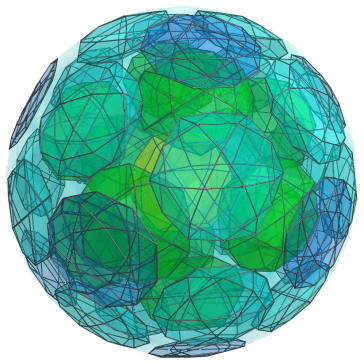## Structure

We shall explore the structure of the cantellated 600-cell by means of its parallel projection into 3D, centered on an icosidodecahedron.

### First Layer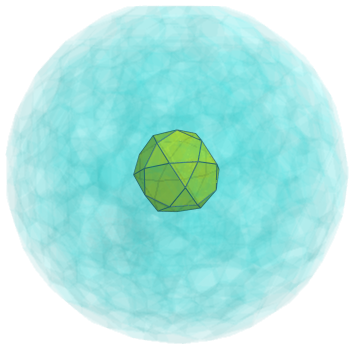The above image shows the nearest icosidodecahedron to the 4D viewpoint. For clarity, we omit all the remaining edges and vertices, and render the other cells in a light transparent color.

### Second Layer

This nearest icosidodecahedron is surrounded by 12 pentagonal prisms, joined to its pentagonal faces, as seen in the next image: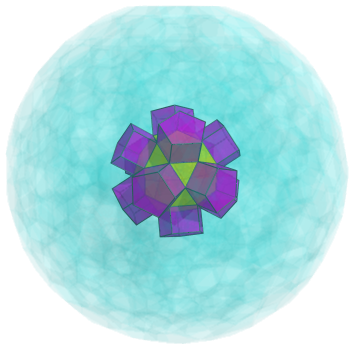The triangular faces of the nearest icosidodecahedron are joined to 20 cuboctahedra, shown below: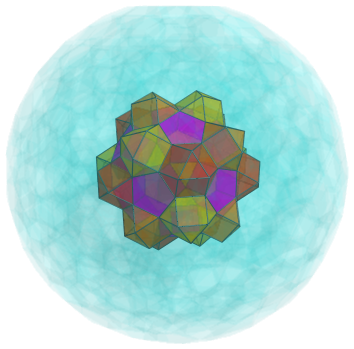### Third Layer

Straddling each adjacent pair of cuboctahedra are another 30 pentagonal prisms. These are shown next: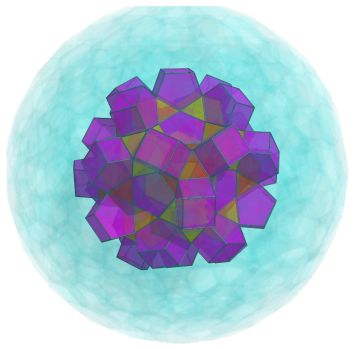Between each trio of prisms are another 20 cuboctahedra: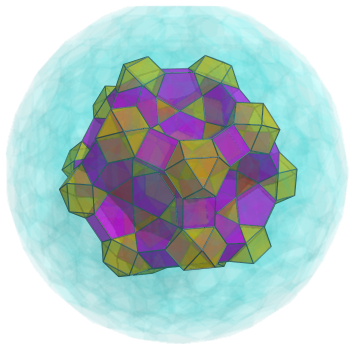The crater-like depressions between these cells are filled in by 12 icosidodecahedra: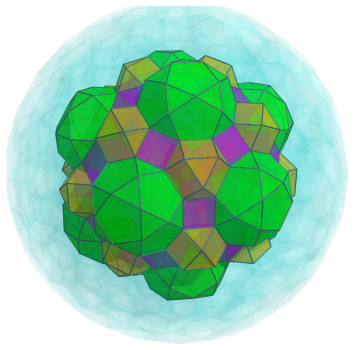### Fourth Layer

The exposed square faces of the pentagonal prisms are joined to another 30 cuboctahedra: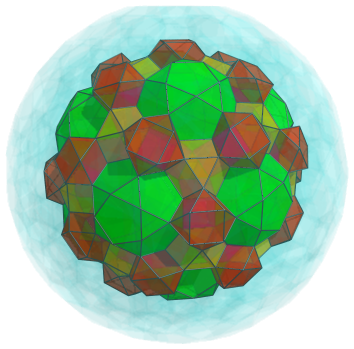Between each adjacent pair of these 30 cuboctahedra is another pentagonal prism, resting on the pentagonal faces of the icosidodecahedra. There are 60 of these prisms: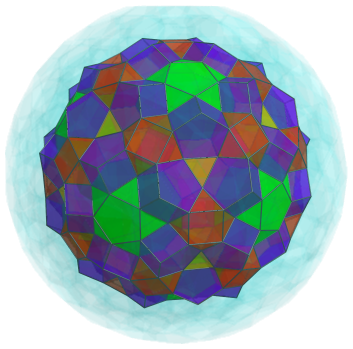These pentagonal prisms form interesting “propellor” configurations around each cuboctahedron.

The exposed pentagonal faces of the icosidodecahedra are connected to another 12 pentagonal prisms, as shown next: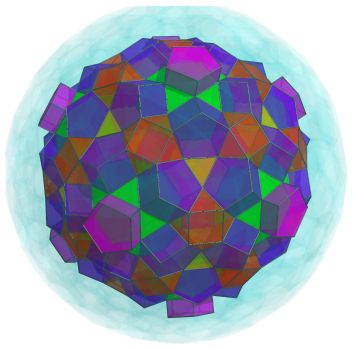The remaining exposed triangular faces of the 12 icosidodecahedra are joined to another 60 cuboctahedra: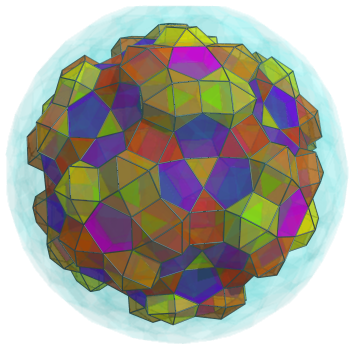Finally, another 20 icosidodecahedra fit into the bowl-shaped depressions above each triplet of pentagonal prisms: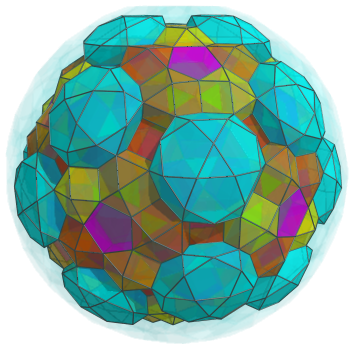### Fifth Layer

The gaps between the 20 icosidodecahedra in the last layer are filled in by pentagonal prisms, 30 of them in total, as shown below: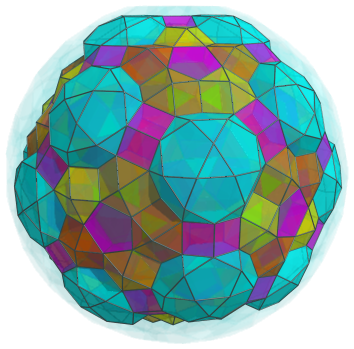Straddling pairs of cuboctahedra in each circle of 5 cuboctahedra are another 60 pentagonal prisms, shown next: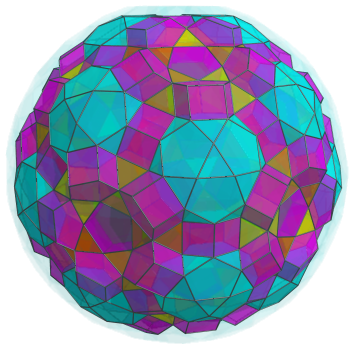The gaps formed by these pentagonal prisms and the previous ones are filled in by more cuboctahedra, 60 of them, occurring in 30 pairs: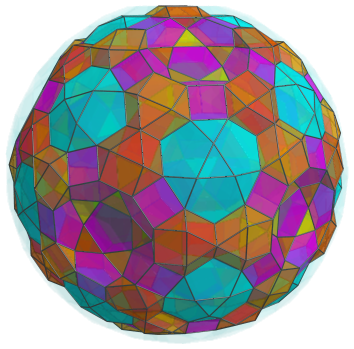The bowl-shaped depressions that remain are now filled by another 12 icosidodecahedra: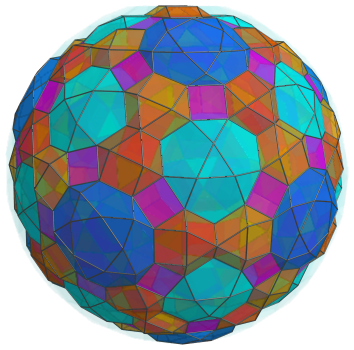The remaining exposed square faces of the pentagonal prisms are joined to yet another 60 cuboctahedra: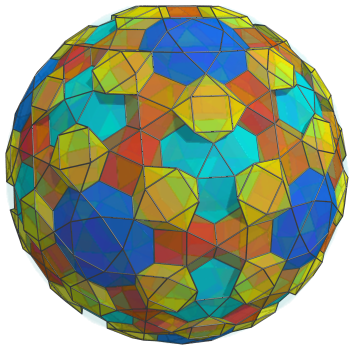The gaps between these cuboctahedra and the pentagonal faces of the cyan icosidodecahedra are obviously filled in by more pentagonal prisms. There are 3 pentagonal prisms per icosidodecahedron, thus totalling 60 more pentagonal prisms: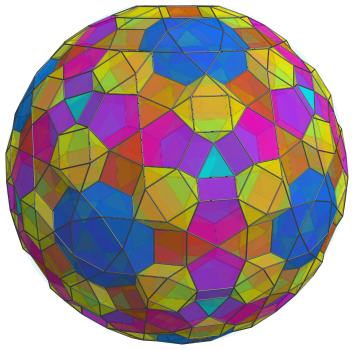The last remaining exposed triangular faces of the cyan icosidodecahedra are joined to another 20 cuboctahedra: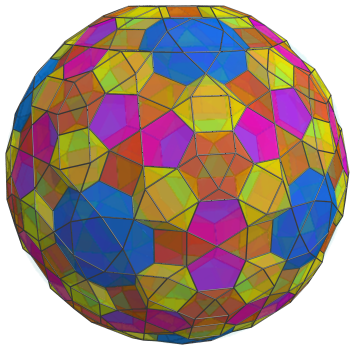Finally, five more pentagonal prisms are joined to each of the blue icosidodecahedra, for a total of another 60 pentagonal prisms before we reach the equator: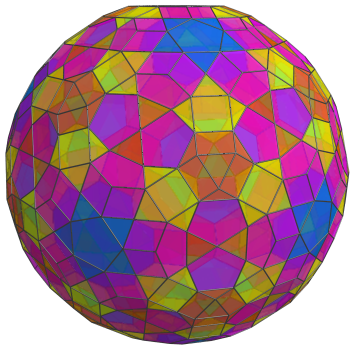These are all the cells that lie on the near side of the cantellated 600-cell. Following these are the cells that lie on its “equator”.

### Equator

At the equator of the cantellated 600-cell are 30 icosidodecahedra, corresponding with the 30 edges of a dodecahedron. The following image shows these cells. For clarity, we omit the other cells we have seen so far.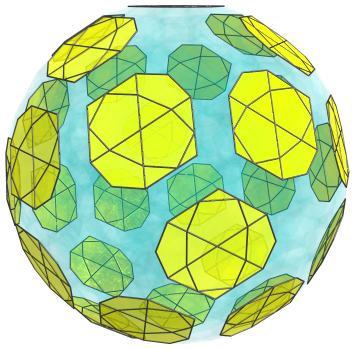These icosidodecahedra are seen from a 90° angle, so they appear as flattened octagons. However, this is merely an artifact of projection; in 4D they are perfectly uniform icosidodecahedra.

There are also 60 cuboctahedra in 12 groups of 5, as seen below: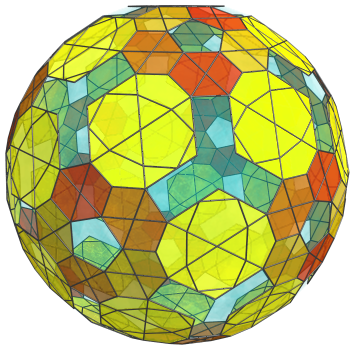These cuboctahedra have been foreshortened into hexagons because they lie at a 90° angle to the 4D viewpoint; in 4D, they are perfectly uniform cuboctahedra.

The pentagonal gaps between these cuboctahedra are filled in by pentagonal prisms: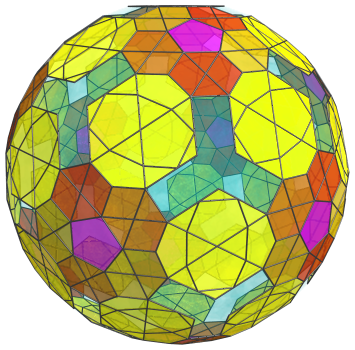These pentagonal prisms are seen at a 90° angle, so they appear flattened into pentagons. Besides these, there are another 60 pentagonal prisms on the equator: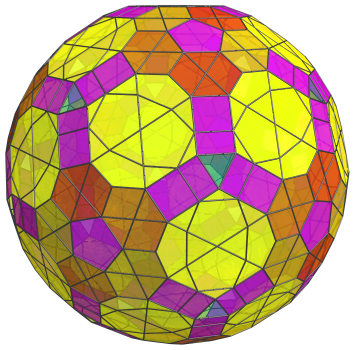These pentagonal prisms are also seen from a 90° angle, but in a different orientation; hence they appear flattened into rectangles. All of these pentagonal prisms are perfectly uniform in 4D.

These are all the cells that lie on the equator of the cantellated 600-cell. The remaining 20 triangular gaps are not images of any cell; rather, they are where 20 cuboctahedra on the near side of the cantellated 600-cell touch their counterparts on the far side.

After this point, we reach the far side of the cantellated 600-cell, where the arrangement of cells exactly mirrors the arrangement we have seen on the near side, with the layers repeating in reverse order.

### Summary

The following table summarizes the cell counts in each of the layers of the cantellated 600-cell:

Region Layer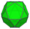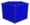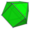Northern hemisphere 1 1 0 0
2 0 12 20
3 12 30 20
4 20 60 + 12 = 72 30 + 60 = 90
5 12 30 + 60 + 60 + 60 = 210 60 + 60 + 20 = 140
Subtotal 45 324 270
Equator 30 12 + 60 = 72 60
Southern hemisphere 5 12 210 140
4 20 72 90
3 12 30 20
2 0 12 20
1 1 0 0
Subtotal 45 324 270
Grand total 120 720 600

## Coordinates

The coordinates of the cantellated 600-cell, centered on the origin and having edge length 2, are all permutations of coordinates and all changes of sign of:

• (0, 0, 2φ, 2+6φ)
• (0, 2, 2φ3, 2φ3)
• (1, 1, 1+4φ, 3+4φ)
• 3, φ3, 1+4φ, 1+4φ)

together with the even permutations of coordinates and all changes of sign of:

 (0, 1, 3φ, φ5) (0, 2φ, 4φ2, 2φ2) (0, 3+2φ, φ4, 3φ2) (1, φ, 2+6φ, φ2) (1, φ, 4φ2, 1+3φ) (1, 2φ, φ5, 2+φ) (1, 2+φ, 2φ3, 3φ2) (1, φ3, 3+4φ, 3+2φ) (φ, 2, φ3, φ5) (φ, 2φ2, 1+4φ, 3φ2) (φ, 1+3φ, 2φ3, 3+2φ) (2, φ2, 3+4φ, φ4) (φ2, 2+φ, φ3, 4φ2) (φ2, 2+φ, 1+4φ, 2φ3) (φ2, 3φ, 3+4φ, 2φ2) (2φ, 2+φ, 1+3φ, 3+4φ) (2φ, 1+3φ, 1+4φ, φ4) (φ3, 3φ, 2φ3, 1+3φ)

where φ=(1+√5)/2 is the Golden Ratio.

Last updated 18 Jun 2019.